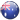# AUS:+61-280-07-5697

The activity of a radioactive element is measured by the rate at which it changes into its daughter element. The rate of radioactive disintegration is independent of temperature, pressure or the state of chemical combination of the element, However, it does depend upon the nature of the element. The rate of nuclear disintegration of radioactive substance is determined by counting the number of particles emitted per unit time by using instruments .like Scintillation Counter, or Geiger-Muller Counter or Wilson cloud chamber.

It has been experimentally observed that in all nuclear disintegration reactions the rate of disintegration (or decay) is directly proportional to the number of atoms present in a sample at that time. Since it refers to the characteristic of a first order reaction. Therefore, radioactive disintegration can be regarded as similar to first order chemical reaction. Consider a radioactive element A disintegrating to give products.

A         à Products

Let N0 be the number of atoms in the beginning at time t= 0

N be the number of atoms of A at time

The rate of disintegration i.e., -dN / dt  at time ‘t’ is directly proportional to N. It can be expressed as

-dN / dt  N

-dN/dt = Λn

where λ is called decay constant or disintegration constant.

Rearrange equation (13.1) and integrate it

-ʃ dN/dt = ʃ λ dt

– in N = λt + c

where C is constant of integration.

Now, when t = 0, number of atoms = N0

– ln N0 = 0 + C or C = – 1n N0

DECAY CONSTANT or DISINTEGRATION CONSTANT (A.)

The definition of decay constant follows from the following mathematical expressions.

(i)                 –dN / dt = λN  or λdt = – dN / N

IF dt is I second , then λ = -Dn/N

HALF-LFE PERIOD

The time required for the decay of the radioactive element to one-half of its original amount is called half-life period. This is usually denoted by t ½  Each radioactive element has its characteristic half-life period. For example, the half-life for the radioactive decay of uranium-238 is 4.51 x 109 years and that of radium-226 is 1.62 x 103 years.

The half-life period (t 1/2)  does not depend upon the initial amount of radioactive element but it depends upon the value of A.. This can be proved as follows:

After time t112, half of the atoms of radioactive substance

disintegrate, so that N = N0 /2

Substituting in equation (13.3), we have

Log no /N0 /2 = λ ½ / 2.303   or t ½ = 2.303 / λ x log 2

T ½ = 0.693 / λ

Since λ is a constant, the time required for disintegration of one-half of the original amount of radioactive substance is independent of the initial amount of radioactive substance.

SIGNIFICANCE OF HALF-LIFE PER

(i) Stability of nuclei. The value of half  life period can give some idea regarding the relative stability of radioisotopes. An isotope having longer half-life period is relatively more stable than the isotope with shorter half-life period. For example, 238U has a longer half-life period (4.5 x 109 years) whereas 214Po has a very short half-life period (1.64 x 10-8 seconds).It implies that 214Po is highly unstable whereas 238U is quite stable as far as radioactive decay is concerned.

(ii) Half life period is independent of initial amount of radioactive substance.

(iii) The amount of substance left after n half lives is given by

N = N0(1/2)n

where,             N is amount after n half lives

N0 is the initial amount of radioactive substance